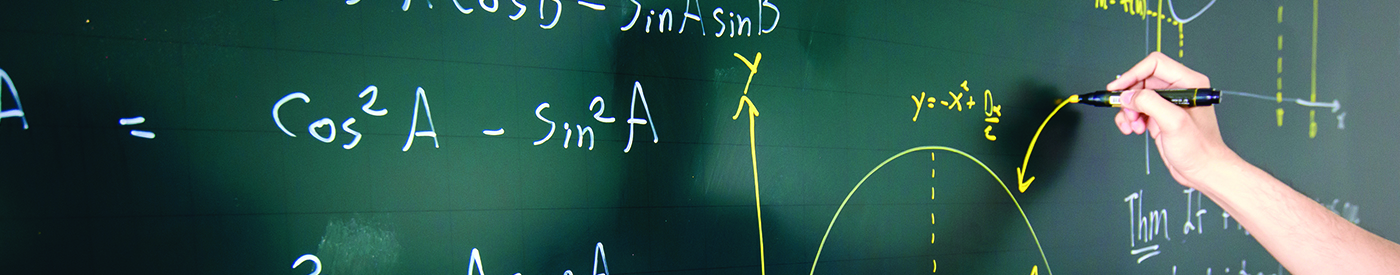## Fusion Math S2 (14 yr old)

##### Objectives
1. Generate greater interest in Mathematics and enhance students’ Mathematical knowledge.
2. Sharpen students’ ingenious problem solving skills and reinforce their abilities to attempt challenging Mathematical problems.
3. Familiarize students with topics in line with SMO (Junior).

Semester 1 (Jan – Mar) Semester 1 (Mar – May)
– Algebra
– Modulus
– Divisibility
– Congruence
– HCF & LCM
– Perfect Square & Prime Number
– Integer Part & Fractional Part
– Arithmetic & Geometric Progression
– Indices & Surds
– A- Diophantine Equation
– Inequalities (Max, Min, AM-GM)
– Geometry (Angles)
– Geometry (Lengths)
– Geometry (Areas)
– Combinatorics
– Revision Papers
Semester 2 (Jun – Sep) Semester 2 (Sep – Nov)
– Introduction to Trigonometry
– Inverse Trigonometric Functions
– Trigonometric Identities
– Cosine & Sine Rules for Triangles
– Triangles